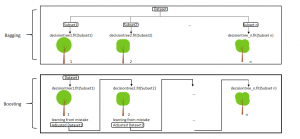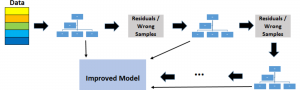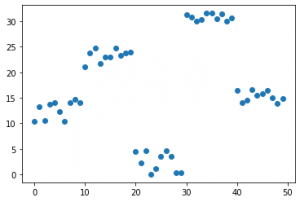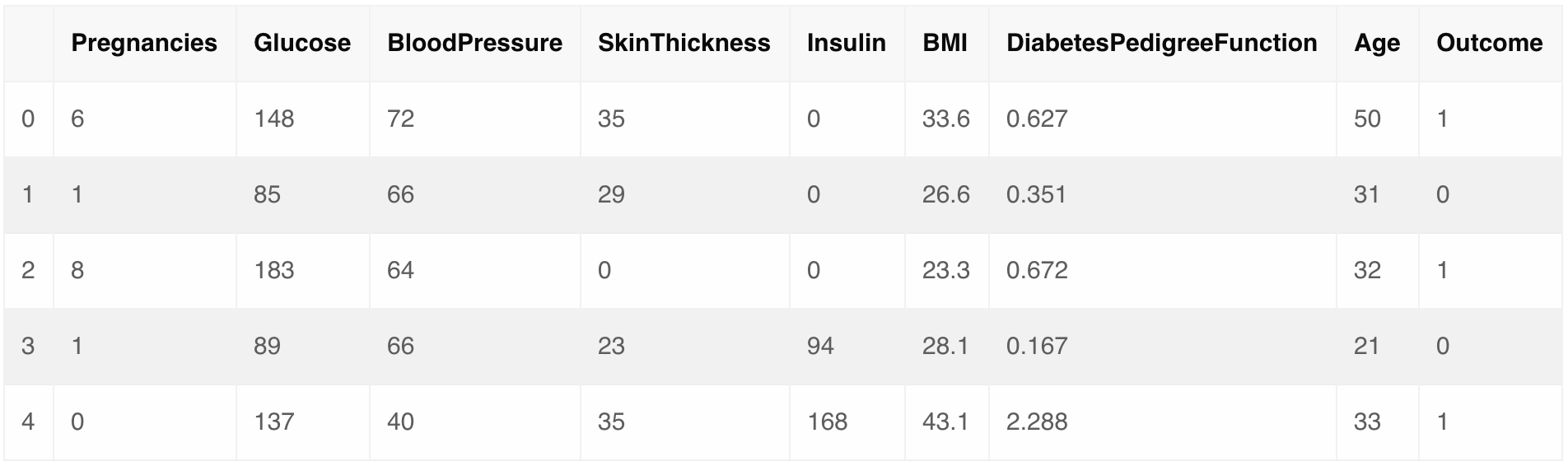# Gradient Boosting – A Concise Introduction from ScratchGradient Boosting is a machine learning algorithm, used for both classification and regression problems. It works on the principle that many weak learners (eg: shallow trees) can together make a more accurate predictor.A Concise Introduction to Gradient Boosting. Photo by Zibik

## How does Gradient Boosting Works?

Gradient boosting works by building simpler (weak) prediction models sequentially where each model tries to predict the error left over by the previous model.

But, what is a weak learning model? A model that does slightly better than random predictions is a weak learner.

I will show you the exact formula shortly.

But for clearly understanding the underlying principles and working of GBT, it’s important to first learn the basic concept of ensemble learning.

This tutorial will take you through the concepts behind gradient boosting and also through two practical implementations of the algorithm:

2. Using the scikit-learn in-built function.

## Contents

1. Ensemble Learning
2. Why boosting?
• Learning rate and n_estimators (hyperparameters)
• Algorithm
5. Implementation
• Implementation from scratch
• Implementation using scikit-learn
6. Improving model perfomance
• Shrinkage
• Regularization
• Tree Constraints
7. References

## Ensemble Learning

Ensemble learning, in general, is a model that makes predictions based on a number of different models. By combining a number of different models, an ensemble learning tends to be more flexible (less bias) and less data sensitive (less variance).

The two most popular ensemble learning methods are bagging and boosting.

1. Bagging : Training a bunch of models in parallel way. Each model learns from a random subset of the data, where the dataset is same size as original but is randomly sampled with replacement (bootstrapped).
2. Boosting : Training a bunch of models sequentially. Each model learns from the mistakes of the previous model. That is, the subsequent models tries to explain and predict the error left over by the previous model.The application of bagging is found in Random Forests. Random forests are a parallel combination of decision trees. Each tree is trained on random subset of the same data and the results from all trees are averaged to find the classification.

The application of boosting is found in Gradient Boosting Decision Trees, about which we are going to discuss in more detail.

## Why Boosting?

Boosting works on the principle of improving mistakes of the previous learner through the next learner.

In boosting, weak learners (ex: decision trees with only the stump) are used which perform only slightly better than a random chance. Boosting focuses on sequentially adding up these weak learners and filtering out the observations that a learner gets correct at every step. Basically, the stress is on developing new weak learners to handle the remaining difficult observations at each step.

One of the very first boosting algorithms developed was Adaboost. Gradient boosting improvised upon some of the features of Adaboost to create a stronger and more efficient algorithm.

Let’s look at a brief overview of Adaboost.

Adaboost uses decision stumps as weak learners. Decision stumps are nothing but decision trees with only one single split. It also attached weights to observations, adding more weight to ‘difficult-to-classify’ observations and less weight to those that are easy to classify.

The aim is to put stress on the difficult to classify instances for every new weak learner.

So, for the next subsequent model, the misclassified observations will receive more weight, as a result, in the new dataset these observations are sampled more number of times according to their new weights, giving the model a chance to learn more of such records and classify them correctly.

As a result, misclassifying the ‘difficult-to-classify’ would be discouraged.

How?

Gradient boosting simply tries to explain (predict) the error left over by the previous model. And since the loss function optimization is done using gradient descent, and hence the name gradient boosting.

Further, gradient boosting uses short, less-complex decision trees instead of decision stumps.

To understand this in more detail, let’s see how exactly a new weak learner in gradient boosting algorithm learns from the mistakes of previous weak learners.

In gradient boosting decision trees, we combine many weak learners to come up with one strong learner. The weak learners here are the individual decision trees.
All the trees are connected in series and each tree tries to minimize the error of the previous tree.

Due to this sequential connection, boosting algorithms are usually slow to learn (controllable by the developer using the learning rate parameter), but also highly accurate. In statistical learning, models that learn slowly perform better.

The weak learners are fit in such a way that each new learner fits into the residuals of the previous step so as the model improves. The final model adds up the result of each step and thus a stronger learner is eventually achieved.A loss function is used to detect the residuals. For instance, mean squared error (MSE) can be used for a regression task and logarithmic loss (log loss) can be used for classification tasks. It is worth noting that existing trees in the model do not change when a new tree is added. The added decision tree fits the residuals from the current model.

### Understanding the Hyperparameters: Learning rate and n_estimators

Hyperparameters are key parts of learning algorithms which effect the performance and accuracy of a model. The Learning rate and n_estimators are two critical hyperparameters for gradient boosting decision trees.

Learning rate, denoted as α, controls how fast the model learns. This is done by multiplying the error in previous model with the learning rate and then use that in the subsequent trees. So, the lower the learning rate, the slower the model learns.

Each tree added modifies the overall model.

The advantage of slower learning rate is that the model becomes more robust and efficient and avoids overfitting. In statistical learning, models that learn slowly perform better. However, learning slowly comes at a cost.

It takes more time to train the model which brings us to the other significant hyperparameter.

n_estimator is the number of trees used in the model. If the learning rate is low, we need more trees to train the model. However, we need to be very careful at selecting the number of trees. It creates a high risk of overfitting to use too many trees.

### Note

One problem that we may encounter in gradient boosting decision trees but not random forests is overfitting due to the addition of too many trees. In random forests, the addition of too many trees won’t cause overfitting. The accuracy of the model doesn’t improve after a certain point but no problem of overfitting is faced.

On the other hand, in gradient boosting decision trees we have to be careful about the number of trees we select, because having too many weak learners in the model may lead to overfitting of data.

Therefore, gradient boosting decision trees require very careful tuning of the hyperparameters.

Till now, we have seen how gradient boosting works in theory. Now, we will dive into the maths and logic behind it so that everything is very clear. Let’s discuss the algorithm step-by-step and make a python program that applies this algorithm to real-time data.

First, let’s go over the basic principle behind gradient boosting once again.

Your main aim is to predict a y given a set of x. The difference between the prediction and the actual value is known as the residual (or in this case, pseudo residuals), on the basis of which the gradient boosting builds successive trees. We know this.

### The Algorithm

Let’s say the output model y when fit to only 1 decision tree, is given by:

$$y = A_1 + (B_1*X) + e_1$$

where, e_1 is the residual from this decision tree.

In gradient boosting, we fit the consecutive decision trees on the residual from the last one. So when gradient boosting is applied to this model, the consecutive decision trees will be mathematically represented as:

$$e_1 = A_2 + (B_2 * X) + e_2$$

and

$$e_2 = A_3 + (B_3 * X) + e_3$$

Note that here we stop at 3 decision trees, but in an actual gradient boosting model, the number of learners or decision trees is much more.

Combining all three equations, the final model of the decision tree will be given by:

$$y = A_1 + A_2 + A_3 + (B_1 * x) + (B_2 * x) + (B_3 * x) + e_3$$

Let’s consider simulated data as shown in scatterplot below with 1 input (x) and 1 output (y) variables.# The above plot has been generated using the following code
x = np.arange(0, 50)
x = pd.DataFrame({'x': x})

# just random uniform distributions in different range

y1 = np.random.uniform(10, 15, 10)
y2 = np.random.uniform(20, 25, 10)
y3 = np.random.uniform(0, 5, 10)
y4 = np.random.uniform(30, 32, 10)
y5 = np.random.uniform(13, 17, 10)

y = np.concatenate((y1, y2, y3, y4, y5))
y = y[:, None]

1. Fit a decision tree on data. [call x as input and y as output]
xi = x  # initialization of input
yi = y  # initialization of target
# x,y --> use where no need to change original y
ei = 0  # initialization of error
n = len(yi)  # number of rows
predf = 0  # initial prediction 0

for i in range(30):  # loop will make 30 trees (n_estimators).
# DecisionTree scratch code can be found on www.kaggle.com/grroverpr/gradient-boosting-simplified
tree = DecisionTree(xi, yi)
# It just create a single decision tree with provided min. sample leaf
# For selected input variable, this splits (<n and >n) data so that std. deviation of
tree.find_better_split(0)
# target variable in both splits is minimum as compared to all other splits

# finds index where this best split occurs
r = np.where(xi == tree.split)

left_idx = np.where(xi <= tree.split)  # index lhs of split
right_idx = np.where(xi > tree.split)  # index rhs of split

1. Calculate error residuals. Actual target value, minus predicted target value [e1= y – y_predicted1 ]
2. Fit a new model on error residuals as target variable with same input variables [call it e1_predicted]
3. Add the predicted residuals to the previous predictions
[y_predicted2 = y_predicted1 + e1_predicted]
4. Fit another model on residuals that is still left. i.e. [e2 = y – y_predicted2] and repeat steps 2 to 5 until it starts overfitting or the sum of residuals become constant. Overfitting can be controlled by consistently checking accuracy on validation data.
# predictions by ith decisision tree

predi = np.zeros(n)
# replace left side mean y
np.put(predi, left_idx, np.repeat(np.mean(yi[left_idx]), r))
np.put(predi, right_idx, np.repeat(
np.mean(yi[right_idx]), n-r))  # right side mean y

predi = predi[:, None]  # make long vector (nx1) in compatible with y
# final prediction will be previous prediction value + new prediction of residual
predf = predf + predi

ei = y - predf  # needed originl y here as residual always from original y
yi = ei  # update yi as residual to reloop


The code above is a very basic implementation of gradient boosting trees. The actual libraries have a lot of hyperparameters that can be tuned for better results. This can be better understood by using the gradient boosting algorithm on a real dataset.

### Implementation using scikit-learn

For implementation on a dataset, we will be using the PIMA Indians Diabetes dataset, which has information about a an individual’s health parameters and an output of 0 or 1, depending on whether or not he has diabates.
The task here is classify a individual as diabetic, when given the required inputs about his health.

First, let’s import all required libraries.

# Import all relevant libraries
import numpy as np
import pandas as pd
from sklearn.preprocessing import StandardScaler
from sklearn.model_selection import train_test_split
from sklearn.metrics import accuracy_score, confusion_matrix
from sklearn import preprocessing
import warnings
warnings.filterwarnings("ignore")


Now load the dataset and look at the columns to understand the given information better.

<

pre># Load the dataset pima = pd.read_csv('diabetes.csv') pima.head()For training and testing our model, the data has to be divided into train and test data.
We will also scale the data to lie between 0 and 1.

# Split dataset into test and train data
X_train, X_test, y_train, y_test = train_test_split(pima.drop('Outcome', axis=1),
pima['Outcome'], test_size=0.2)

# Scale the data
scaler = preprocessing.StandardScaler().fit(X_train)
X_train_transformed = scaler.transform(X_train)
X_test_transformed = scaler.transform(X_test)


Now that the data has been sufficiently preprocessed, let’s go ahead with defining the Gradient Boosting Classifier along with it’s hyperparameters. Next, we will fit this model on the training data.

# Define Gradient Boosting Classifier with hyperparameters
# Fit train data to GBC
gbc.fit(X_train_transformed, y_train)


GradientBoostingClassifier(criterion='friedman_mse', init=None,
learning_rate=0.05, loss='deviance', max_depth=3,
max_features=5, max_leaf_nodes=None,
min_impurity_decrease=0.0, min_impurity_split=None,
min_samples_leaf=1, min_samples_split=2,
min_weight_fraction_leaf=0.0, n_estimators=500,
n_iter_no_change=None, presort='auto',
random_state=100, subsample=1.0, tol=0.0001,
validation_fraction=0.1, verbose=0,
warm_start=False)


The model has been trained and we can now observe the outputs as well.

Below, you can see the confusion matrix of the model, which gives a report of the number of classifications and misclassifications.

# Confusion matrix will give number of correct and incorrect classifications
print(confusion_matrix(y_test, gbc.predict(X_test_transformed)))


[[82 17]
[25 30]]


The number of misclassifications by the Gradient Boosting Classifier are 42, comapared to 112 correct classifications. The model has performed decently.
Let’s check the accuracy

# Accuracy of model
print("GBC accuracy is %2.2f" % accuracy_score(
y_test, gbc.predict(X_test_transformed)))


GBC accuracy is 0.73


The accuracy is 73%, which is average. This can be improved by tuning the hyperparameters or processing the data to remove outliers.|

The discussion above is just the tip of iceberg when it comes to gradient boosting. The underlying concepts can be understood in more detail by starting with the very basics of machine learning algorithms and understanding the working of python code.
This however gives us the basic idea behind gradient boosting and its underlying working principles.

## Improving performance of gradient boosted decision trees

As we already discussed above, gradient boosting algorithms are prone to overfitting and consequently poor performance on test dataset. There are some pointers you can keep in mind to improve the performance of gradient boosting algorithm.

Stochastic gradient boosting involves subsampling the training dataset and training individual learners on random samples created by this subsampling. This reduces the correlation between results from individual learners and combining results with low correlation provides us with a better overall result.

A few variants of stochastic boosting that can be used:

1. Subsample rows before creating each tree.
2. Subsample columns before creating each tree.
3. Subsample columns before considering each split.

### Shrinkage

The predictions of each tree are added together sequentially. Instead, the contribution of each tree to this sum can be weighted to slow down the learning by the algorithm. This weighting is called a shrinkage or a learning rate.

Using a low learning rate can dramatically improve the performance of your gradient boosting model. Usually a learning rate in the range of 0.1 to 0.3 gives the best results.

Keep in mind that a low learning rate can significantly drive up the training time, as your model will require more number of iterations to converge to a final loss value.

### Regularization

L1 and L2 regularization penalties can be implemented on leaf weight values to slow down learning and prevent overfitting.

Gradient tree boosting implementations often also use regularization by limiting the minimum number of observations in trees’ terminal nodes

### Tree Constraints

There are a number of ways in which a tree can be constrained to improve performance.

1. Number of trees : Adding excessive number of trees can lead to overfitting, so it is important to stop at the point where the loss value converges.
2. Tree depth : Shorter trees are preferred over more complex trees. Limit the number of levels in a tree to 4-8.
3. Minimum improvement in loss : If you have gone over the process of decision making in decision trees, you will know that there is a loss associated at each level of a decision tree. A minimum improvement in loss required to build a new level in a tree can be predecided. This helps in pruning the tree to keep it short.
4. Number of observations per split :This imposes a minimum constraint on the amount of training data at a training node before a split can be consideredReference project template for all your Data Science projects. Learn  how to load the data, get an overview of the data. Perform EDA, prepare data, build models & improve model performance.

## Similar Articles

### Logistic Regression – A Complete Tutorial With Examples in RReference project template for all your Data Science projects. Learn  how to load the data, get an overview of the data. Perform EDA, prepare data, build models & improve model performance.Course Preview

## Machine Learning A-Z™: Hands-On Python & R In Data Science

### Free Sample Videos:#### Machine Learning A-Z™: Hands-On Python & R In Data Science#### Machine Learning A-Z™: Hands-On Python & R In Data Science#### Machine Learning A-Z™: Hands-On Python & R In Data Science#### Machine Learning A-Z™: Hands-On Python & R In Data Science